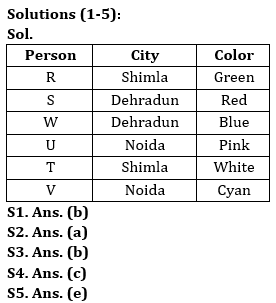Latest Banking jobs   »

# Reasoning Ability Quiz For Bank Foundation 2023 -25th May

Directions (1-5): Answer the following question based on the information given below.
Six people (R, S, T, U, V and W) like different colors among Red, Green, Blue, White, Pink and Cyan. They visit three different cities like Noida, Dehradun, Shimla such that two people visit each city.
The one who likes white does not visit Dehradun. R who likes green, visits Shimla. S and the one who likes blue, visit the same city. U likes pink but does not visit Shimla. T neither likes blue nor visits the same city as U. V likes cyan but does not visit Dehradun.

Q1. W visits which of the following city?
(a) Shimla
(c) Noida
(d) Either Shimla or Noida
(e) None of these

Q2. T likes__________ and visits ________.
(a) White, Shimla
(b) White, Noida
(c) Red, Shimla
(e) None of these

Q3. Who among the following person visits the same city as U?
(a) S
(b) V
(c) W
(d) R
(e) None of these

Q4. Which of the following pair visit Noida?
(a) T, S
(b) T, W
(c) V, U
(d) T, V
(e) None of these

Q5. Which of the following statement is correct?
(a) V likes cyan and visits Shimla
(b) S likes white
(c) W likes blue and visits Noida
(d) T likes red and visits Shimla
(e) U visits Noida

Directions (6-10): In the following questions assuming the given statement to be true, find which of the conclusion(s) among given conclusions is/are definitely true and then give your answers accordingly.

Q6. Statements: K > G = Q > T, L > T < N, N ≥ O
Conclusions: I. K > T
II. O > Q
(a) Only I is true
(b) Only II is true
(c) Either I or II is true
(d) Neither I nor II is true
(e) Both I and II are true

Q7. Statements: K > G = Q > T, L > T < N, N ≥ O
Conclusions: I. G < N
II. T < Q
(a) Only I is true
(b) Only II is true
(c) Either I or II is true
(d) Neither I nor II is true
(e) Both I and II are true

Q8. Statements: K > G > T, L > T < N, N ≥ O, L = Q
Conclusions: I. L > N
II. Q ≤ N
(a) Only I is true
(b) Only II is true
(c) Either I or II is true
(d) Neither I nor II is true
(e) Both I and II are true

Q9. Statement: L < M > R = S < T ≤ U = V = W > X
Conclusions: I. M < V
II. X < U
(a) Only I is true
(b) Only II is true
(c) Either I or II is true
(d) Neither I nor II is true
(e) Both I and II are true

Q10. Statement: F ≥ K < S = Y < M, A > B > E = D > M
Conclusions: I. E > Y
II. K < E
(a) Only I is true
(b) Only II is true
(c) Either I or II is true
(d) Neither I nor II is true
(e) Both I and II are true

SolutionsSolutions (6-10):
S6. Ans. (a)
Sol. I. K > T – True
II. O > Q – False

S7. Ans. (b)
Sol. I. G < N – False
II. T < Q – True

S8. Ans. (c)
Sol. I. L > N – False
II. Q ≤ N – False

S9. Ans. (b)
Sol. I. M < V – False
II. X < U – True

S10. Ans. (e)
Sol. I. E > Y – True
II. K < E – True## FAQs

### What is the selection process of the Bank Clerk?

The selection process of the Bank Clerk is Prelims & Mains.

#### Congratulations!Union Budget 2023-24: Free PDF